Updating search results...

# 57 Results

View
Selected filters:
• Differential EquationsConditional Remix & Share Permitted
CC BY-NC-SA
Rating
5.0 stars

Some of the topics that this book addresses are: Vector spaces; finite-dimensional vector spaces; differential calculus; compactness and completeness; scalar product space; differential equations; multilenear functionals; integration; differentiable manifolds; integral calculus on manifolds; exterior calculus.

Note: this is a 57 MB PDF Document.

Subject:
Calculus
Material Type:
Textbook
Provider:
Harvard University
Provider Set:
Individual Authors
Author:
Sternberg Shlomo and Lynn Loomis
02/16/2011Unrestricted Use
CC BY
Rating
0.0 stars

This contemporary calculus course is the second in a three-part sequence. In this course students continue to explore the concepts, applications, and techniques of Calculus - the mathematics of change. Calculus has wide-spread application in science, economics and engineering, and is a foundation college course for further work in these areas. This is a required class for most science and mathematics majors.Login: guest_oclPassword: ocl

Subject:
Calculus
Material Type:
Full Course
Homework/Assignment
Lecture Notes
Lesson Plan
Syllabus
Provider:
Washington State Board for Community & Technical Colleges
Provider Set:
Open Course Library
10/31/2011Unrestricted Use
CC BY
Rating
5.0 stars

Calculus is designed for the typical two- or three-semester general calculus course, incorporating innovative features to enhance student learning. The book guides students through the core concepts of calculus and helps them understand how those concepts apply to their lives and the world around them. Due to the comprehensive nature of the material, we are offering the book in three volumes for flexibility and efficiency. Volume 2 covers integration, differential equations, sequences and series, and parametric equations and polar coordinates.

Subject:
Calculus
Material Type:
Textbook
Provider:
Rice University
Provider Set:
OpenStax College
Author:
Alfred K. Mulzet
Catherine Abbott
David McCune
David Smith
David Torain
Edwin Jed Herman
Elaine A. Terry
Erica M. Rutter
Gilbert Strang
Joseph Lakey
Joyati Debnath
Julie Levandosky
Kirsten R. Messer
Michelle Merriweather
Nicoleta Virginia Bila
Sheri J. Boyd
Valeree Falduto
02/01/2016Conditional Remix & Share Permitted
CC BY-NC-SA
Rating
0.0 stars

Our writing is based on three premises. First, life sciences students are motivated by and respond well to actual data related to real life sciences problems. Second, the ultimate goal of calculus in the life sciences primarily involves modeling living systems with difference and differential equations. Understanding the concepts of derivative and integral are crucial, but the ability to compute a large array of derivatives and integrals is of secondary importance. Third, the depth of calculus for life sciences students should be comparable to that of the traditional physics and engineering calculus course; else life sciences students will be short changed and their faculty will advise them to take the 'best' (engineering) course.

Subject:
Calculus
Material Type:
Textbook
Provider:
University of Minnesota
Provider Set:
University of Minnesota Libraries Publishing
Author:
James L. Cornette
Ralph A. Ackerman
01/01/2012Unrestricted Use
CC BY
Rating
0.0 stars

This module consists of four units, namely Introduction to Ordinary differential equations, higher order ordinary differential equations, power series solutions and special functions and partial differential equations and their applications. In unit one both first and second order equations are discussed and their solutions obtained with a variety of techniques. in unit two both homogeneous and non-homogeneous higher order equations are considered and a variety of solution techniques are discussed. Some of these techniques include the variation of parameters, the method of undetermined coefficients and the inverse operators. In unit three series solutions of differential equations are discussed. Also discussed special functions of mathematical physics. In unit four we look at partial differential equations and their solution by separation of variables.Other topics discussed are Laplace transforms, Fourier series, Fourier transforms and their applications.

Subject:
Mathematics
Material Type:
Module
Provider:
African Virtual University
Provider Set:
OER@AVU
Author:
George L. Ekol
03/15/2018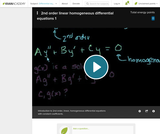Conditional Remix & Share Permitted
CC BY-NC-SA
Rating
0.0 stars

This 10-minute video lesson provides an introduction to 2nd order, linear, homogeneous differential equations with constant coefficients. [Differential Equations playlist: Lesson 13 of 45]

Subject:
Algebra
Material Type:
Lecture
Provider:
Provider Set:
Author:
Khan, Salman
02/20/2011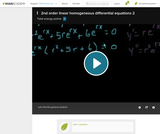Conditional Remix & Share Permitted
CC BY-NC-SA
Rating
0.0 stars

This 8-minute video lesson continues the discussion from the previous video and shows how to find the general solution. [Differential Equations playlist: Lesson 14 of 45]

Subject:
Algebra
Material Type:
Lecture
Provider:
Provider Set:
Author:
Khan, Salman
02/20/2011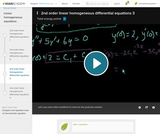Conditional Remix & Share Permitted
CC BY-NC-SA
Rating
0.0 stars

This 6-minute video lesson continues the discussion from the previous videos and introduces some initial conditions to solve for the particular solution. [Differential Equations playlist: Lesson 15 of 45]

Subject:
Algebra
Material Type:
Lecture
Provider:
Provider Set:
Author:
Khan, Salman
02/20/2011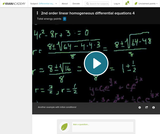Conditional Remix & Share Permitted
CC BY-NC-SA
Rating
0.0 stars

This 9-minute video lesson continues the discussion from the previous videos and provides another example using initial conditions. [Differential Equations playlist: Lesson 16 of 45]

Subject:
Algebra
Material Type:
Lecture
Provider:
Provider Set:
Author:
Khan, Salman
02/20/2011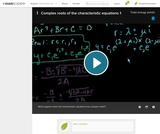Conditional Remix & Share Permitted
CC BY-NC-SA
Rating
0.0 stars

This 10-minute video lesson looks at what happens when the characteristic equations has complex roots. [Differential Equations playlist: Lesson 17 of 45]

Subject:
Algebra
Material Type:
Lecture
Provider:
Provider Set:
Author:
Khan, Salman
02/20/2011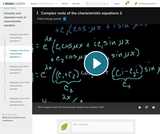Conditional Remix & Share Permitted
CC BY-NC-SA
Rating
0.0 stars

This 10-minute video lesson continues the discussion of what happens when the characteristic equation has complex roots. [Differential Equations playlist: Lesson 18 of 45]

Subject:
Algebra
Material Type:
Lecture
Provider:
Provider Set:
Author:
Khan, Salman
02/20/2011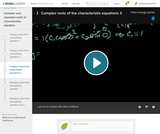Conditional Remix & Share Permitted
CC BY-NC-SA
Rating
0.0 stars

This 10-minute video lesson concludes the series on complex roots of characteristic equations and demonstrates an example with initial conditions. [Differential Equations playlist: Lesson 19 of 45]

Subject:
Algebra
Material Type:
Lecture
Provider:
Provider Set:
Author:
Khan, Salman
02/20/2011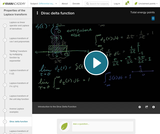Conditional Remix & Share Permitted
CC BY-NC-SA
Rating
0.0 stars

This 18-minute video lesson provides an introduction to the Dirac Delta Function. [Differential Equations playlist: Lesson 41 of 45]

Subject:
Algebra
Material Type:
Lecture
Provider:
Provider Set:
Author:
Khan, Salman
08/01/2011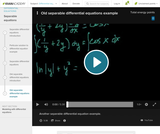Conditional Remix & Share Permitted
CC BY-NC-SA
Rating
0.0 stars

This 12-minute video lesson gives the first example of solving an exact differential equation. [Differential Equations playlist: Lesson 6 of 45]

Subject:
Algebra
Material Type:
Lecture
Provider:
Provider Set:
Author:
Khan, Salman
02/20/2011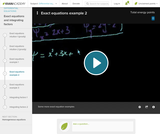Conditional Remix & Share Permitted
CC BY-NC-SA
Rating
0.0 stars

This 8-minute video lesson provides some more examples of exact equations. [Differential Equations playlist: Lesson 7 of 45]

Subject:
Algebra
Material Type:
Lecture
Provider:
Provider Set:
Author:
Khan, Salman
02/20/2011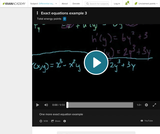Conditional Remix & Share Permitted
CC BY-NC-SA
Rating
0.0 stars

This 10-minute vidoe lesson provides one more exact equation example [Differential Equations playlist: Lesson 8 of 45]

Subject:
Algebra
Material Type:
Lecture
Provider:
Provider Set:
Author:
Khan, Salman
02/20/2011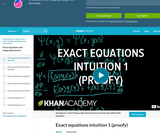Conditional Remix & Share Permitted
CC BY-NC-SA
Rating
0.0 stars

This 10-minute video lesson provides intuiton on exact equations including the chain rule using partial derivatives (not a proof; more intuition). [Differential Equations playlist: Lesson 4 of 45]

Subject:
Algebra
Material Type:
Lecture
Provider:
Provider Set:
Author:
Salman Khan
02/20/2011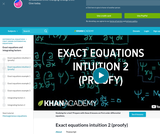Conditional Remix & Share Permitted
CC BY-NC-SA
Rating
0.0 stars

This 11-minute video lesson provides more intuitive building blocks for exact equations. [Differential Equations playlist: Lesson 5 of 45]

Subject:
Algebra
Material Type:
Lecture
Provider:
Provider Set:
Author:
Salman Khan
02/20/2011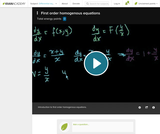Conditional Remix & Share Permitted
CC BY-NC-SA
Rating
0.0 stars

This 7-minute video lesson provides an introduction to first order homogenous equations. [Differential Equations playlist: Lesson 11 of 45]

Subject:
Algebra
Material Type:
Lecture
Provider:
Provider Set:
Author:
Khan, Salman
02/20/2011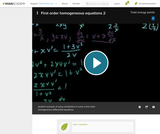Conditional Remix & Share Permitted
CC BY-NC-SA
Rating
0.0 stars

This 8-minute video lesson provides another example of using substitution to solve a first order homogeneous differential equations. [Differential Equations playlist: Lesson 12 of 45]

Subject:
Algebra
Material Type:
Lecture
Provider: